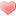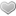Hello everybody, here we are today with Wordful, new exciting quiz for Android, which is on our review and find solutions. The game has a very simple operation: you must be able to connect the word using the letters on the screen. The app was built by Smart Up Inc. Use the search form below to find your answer. Enter 3 letters from the first row. You can also search daily puzzles here.

## Enter first three letters from the first row:

S R G E
E S I L
O H C N
O Z T A
D C E D
E R G I
S K S E
U I F R
S C E R
O P R O
H U M R
T O M I
P R A E
C E F M
A E E U
M R A S
S I C E
Z E O L
N O C I
T U M S
G U R I
H R E D
C N R E
Y A V B
R S N R
P I N E
T M C E
A P A B
A F A C
S L A E
L S R Y
E S O N
E X Y I
A R D E
O M L L
S E W O
T Y S N
S E P U
T A M T
I E N F
S O R P
T E T S
A E I O
G D P H
I R X T
A Y A L
C H L U
G A F A
F W O M
O B O C
O E N E
T D R A
H K T N
T O C O
U O T O
M C C L
A F D O
F P L W
L I L E
E P E R
N T R E
H A A B
P L R E
E E D E
E O L M
H W G U
P Y M N
E N R E
Z D U K
E T E R
B A O A
R C L M
M O N R
O B P I
L U M C
R E B E
N I S E
K S T N
A R E N
A M E C(414 votes, average: 2,90 out of 5)Loading...

### Last puzzle searches:

ysw+&, prs'v, mrs', Ckl++, E+I+M, Doidr, Cgsec, Ttr'&, '&, Zpi'&, AspA=, Ckl+, &, prs.), prs(,, prs,), &, CGI'+, yce&a, prs.)
WordFul.info is not affiliated with the applications mentioned on this site. All intellectual property, trademarks, and copyrighted material is property of their respective developers.